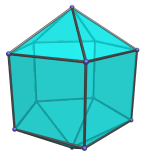# The Elongated Pentagonal Bipyramid

The elongated pentagonal bipyramid is the 16th Johnson solid (J16). It has 12 vertices, 25 edges, and 15 faces (10 equilateral triangles and 5 squares).The elongated pentagonal bipyramid can be constructed by attaching two pentagonal pyramids to a pentagonal prism. Its name describes its relation to the pentagonal bipyramid: inserting a pentagonal prism between the two constituent pentagonal pyramids to make the bipyramid longer.

## Projections

Here are some views of the elongated pentagonal bipyramid from various angles:

Projection Envelope DescriptionRegular pentagon

Top view.Hexagon

Front view.Hexagon

Side view.

## Coordinates

The Cartesian coordinates of the elongated pentagonal bipyramid with edge length 2 are:

• (0, 0, ±(1+√((10−2√5)/5)))
• (√((10+2√5)/5), 0, ±1)
• (√((5−√5)/10), ±φ, ±1)
• (−√((5+2√5)/5), ±1, ±1)

where φ=(1+√5)/2 is the Golden Ratio.

## Occurrences

The elongated pentagonal bipyramid occurs as cells in the following CRF polytopes:

Last updated 18 Jun 2019.